Courses

# Operators and Commutators - General Formalism of Wave Mechanics, Quantum Mechanics, CSIR-NET Physics Notes | EduRev

## Physics for IIT JAM, UGC - NET, CSIR NET

Created by: Akhilesh Thakur

## Physics : Operators and Commutators - General Formalism of Wave Mechanics, Quantum Mechanics, CSIR-NET Physics Notes | EduRev

The document Operators and Commutators - General Formalism of Wave Mechanics, Quantum Mechanics, CSIR-NET Physics Notes | EduRev is a part of the Physics Course Physics for IIT JAM, UGC - NET, CSIR NET.
All you need of Physics at this link: Physics

Why Operators?

When we work with the Schrödinger Equation, or in any other formulation of Quantum Mechanics, exact values of properties can not be found. Instead we use operators.

The simplest way of expressing Schrödinger's Equation is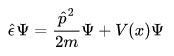Showing that energy = kinetic + potential.

Now this could look deceptively simple if we didn't use operators for energy and momentum. We could simply divide by the wave function Ψ. But like most things, it's never simple. The energy operator acts on the wave function, as does the momentum operator. So we need to find the wave function in order to make any sense of this equation.

A Look at a Few Common Operators

Although we could theoretically come up with an infinite number of operators, in practice there are a few which are much more important than any others.

Momentum

The Momentum operator is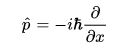So the relative part of the Schrödinger Equation will become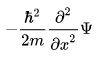Energy

Energy Operator is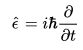So Schrödinger's now looks like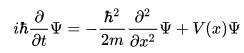As you can see this is now a differential equation, which may or may not be easily solved depending on the potential V(x).
Note that this is the one dimensional form of Schrödinger's Equation, it does become more complex for higher dimensions.

Hamiltonian

We often call the Right Hand Side of this equation the Hamiltonian Operator.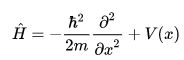and it represents the total energy of the particle of mass m in the Potential Field V.

Expectation Values

In Quantum Mechanics, everything is probabilistic (e.g., the probability of finding a particle is the square of the amplitude of the wave function). So we often want to know the expected value of position, momentum, or anything else, and there is quite a nice method of doing this.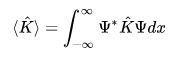So, for instance, if you knew the wave function and wanted to find the expectation value of momentum you'd use the equation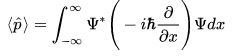Dirac Notation (Bra-Ket Notation)

To simplify notation Paul Dirac came up with a new way of writing states: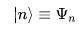i.e a ket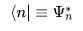i.e. a bra

So the expression for the expectation value of momentum can now be written as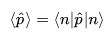This notation is very widely used. It is extremely useful in finite dimensional problems, represented by matrices and state vectors. In 2D, qubits are used (most used in Quantum Information Theory), where: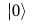and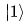are the state vectors.

Commutators

"And you know why four minus one plus ten is fourteen minus one? 'Cause addition is commutative, right." --Tom Lehrer

Commutators are very important in Quantum Mechanics. As well as being how Heisenberg discovered the Uncertainty Principle, they are often used in particle physics. It is known that you cannot know the value of two physical values at the same time if they do not commute.

Mathematical Definition of Commutator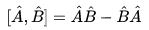This is equal to 0 if they commute and something else if they don't.

As you can probably see all natural numbers will commute. For instance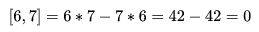But, if we look at Momentum and Position, things start to get interesting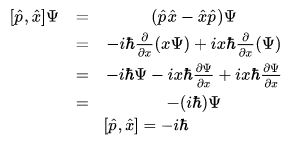Essentially, this implies that you cannot simultaneously know position and momentum precisely for a given moment in time.

However, if we look at the commutator between momentum and energy,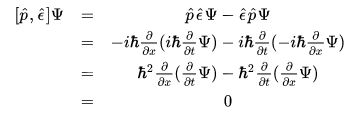From this, we know that momentum and energy commute. Thus, we can find simultaneous eigenfunctions of energy and momentum with definite values of the two observables.

159 docs

,

,

,

,

,

,

,

,

,

,

,

,

,

,

,

,

,

,

,

,

,

,

,

,

,

,

,

;